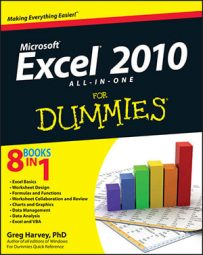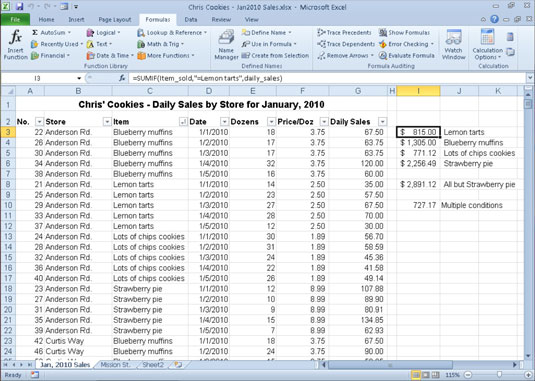##### Excel 2010 All-in-One For DummiesIn Excel 2010, you use the very familiar SUM function to total values in your worksheets. Use the SUMIF function when you want to total only the numbers in a range that meet criteria you specify. These functions are located with the Math & Trig functions on the Ribbon's Formulas tab or in the Select a Category list in the Insert Function dialog box.

## SUM

Excel provides an AutoSum command button on the Home tab of the Ribbon that you can use to quickly build your SUM formulas. You should, however, be aware of the workings of the basic SUM function that the AutoSum button enables you to use so easily.

For the record, the syntax of the SUM function is as follows:

`=SUM(<i>number1</i>,[<i>number2</i>],[...])`

When using the SUM function, only the number1 argument is required; this is the range of numbers in a cell range or array constant that you want added together. You can enter up to a total of 29 other optional number arguments, separated by a commas, in a single SUM formula. For example, you can build a SUM formula that totals numbers in several different ranges like this:

`=SUM(B3:B10,Sheet2!B3:B10,Sheet3!B3:B10)`

In this example, Excel sums the values in the cell range B3:B10 on Sheet1 (the current sheet), Sheet2, and Sheet3 of the workbook, giving you the grand total of all these values.

## SUMIF

What about those times when you want the total of only certain items within a cell range? For those situations, you can use the SUMIF function. The SUMIF function enables you to tell Excel to add together the numbers in a particular range only when those numbers meet the criteria that you specify. The syntax of the SUMIF function is as follows:

`=SUMIF(range,criteria,[sum_range])`

In the SUMIF function, the range argument specifies the range of cells that you want Excel to evaluate when doing the summing; the criteria argument specifies the criteria to be used in evaluating whether to include certain values in the range in the summing; and finally, the optional sum_range argument is the range of all the cells to be summed together. If you omit the sum_range argument, Excel sums only the cells specified in the range argument (and, of course, only if they meet the criteria specified in the criteria argument).

The figure illustrates how you can use the SUMIF function to total sales by the items sold. This figure shows a Sales list sorted by the store location (there are three locations: Anderson Rd., Curtis Way, and Mission Street) and then the item sold. To total the sales of Lemon tarts at all three locations, you could place the following SUMIF formula in cell I3:`=SUMIF(C3:C100,"=Lemon tarts",G3:G62)`

In this example, the cell range C3:C62 contains the list of each item that has been sold in the first five days of January, 2010 (Lemon tarts, Blueberry muffins, Lots of chips cookies, or Strawberry pie). The cell range G3:G62 contains the extended sales made at each store for each item.

The SUMIF formula in cell I3 looks for each occurrence of "Lemon tarts" (the criteria argument for the SUMIF function) in the Item column of the Cookie Sales list and then adds its extended sales price from the Daily Sales column to the total.

The formulas in cells I4, I5, and I6 contain SUMIF functions very similar to the one in cell I3, except that they substitute the name of the dessert goodie in question in place of the "=Lemon tarts" criteria argument. The formula in cell I8, however, is slightly different: This formula sums the sales for all items except for Strawberry pies. It does this with the SUMIF function in the following formula:

`=SUMIF(C3:C62,"<>Strawberry pie",G3:G62)`

Because the item Strawberry pie is preceded with the not operator (<>), Excel sums the daily sale for every item except for strawberry pie.